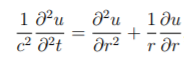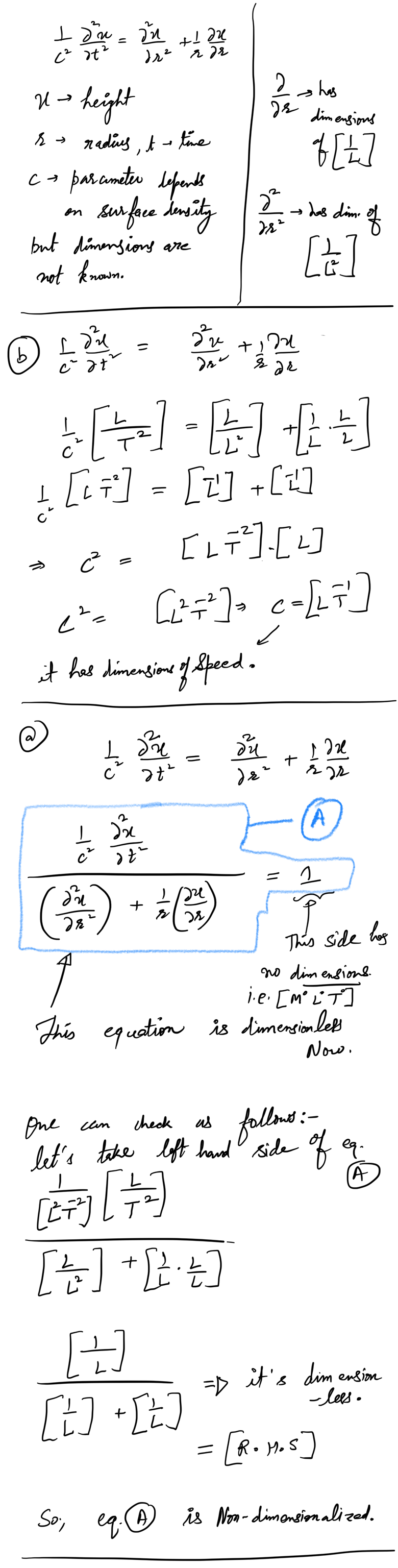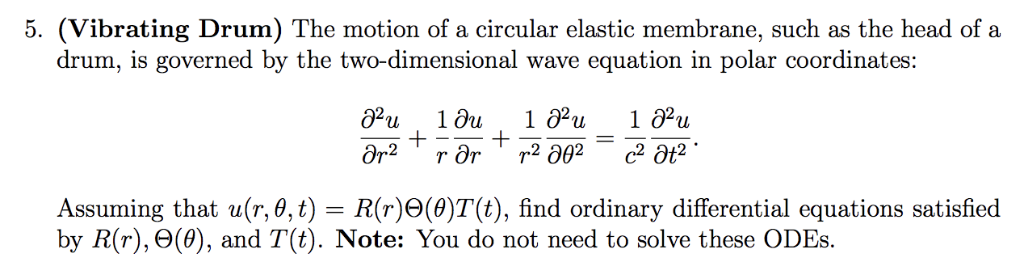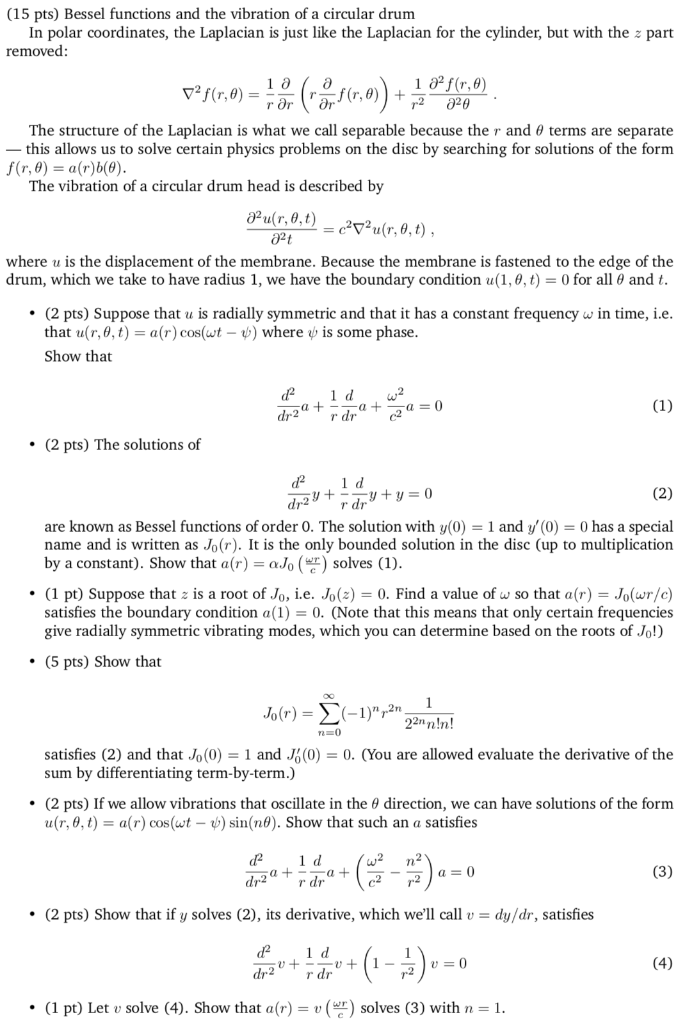# The following model determines the vibration of a drum, specifically the vibration of the batter head,...

The following model determines the vibration of a drum, specifically the vibration of the batter head, where the wave equation is solved in polar coordinates.u(r,t) is the height of a drum's surface at radius (r) and time (t), which is relative to its position at rest.

c is a parameter which depends on surface density (kg//m3) of the drum head, and with tension t (newtons) applied to it.

Q1. Non-dimensionalise the following equation

Q2. From the question, what dimension should c have?

1 д? 1ди д?u дr2 + с? д?t т де##### Add Answer of: The following model determines the vibration of a drum, specifically the vibration of the batter head,...
Similar Homework Help Questions
• ### 5. (Vibrating Drum) The motion of a circular elastic membrane, such as the head of a drum, is gov...5. (Vibrating Drum) The motion of a circular elastic membrane, such as the head of a drum, is governed by the two-dimensional wave equation in polar coordinates: tions satisfied by R(r), e(6), and T(t). Note: You do not need to solve these ODEs. 5. (Vibrating Drum) The motion of a circular elastic membrane, such as the head of a drum, is governed by the two-dimensional wave equation in polar coordinates: tions satisfied by R(r), e(6), and T(t). Note: You do...

• ### (15 pts) Bessel functions and the vibration of a circular drum In polar coordinates, the Laplacia...(15 pts) Bessel functions and the vibration of a circular drum In polar coordinates, the Laplacian is just like the Laplacian for the cylinder, but with the removed part เอ The structure of the Laplacian is what we call separable because the r and 0 terms are separate this allows us to solve certain physics problems on the disc by searching for solutions of the form f(r,0)-ar)b() The vibration of a circular drum head is described by 02t where u...

Free Homework App